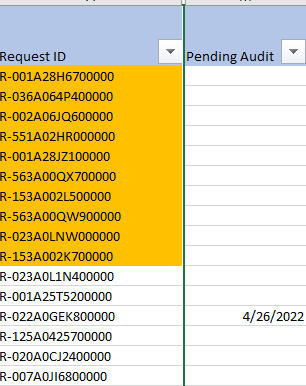cancel
Showing results for
Did you mean:Helper V

## Counting blanks

Hello,

Need help with the conditional function like where. I want to count requestID where pending audit = blanks.

OMPending QA =
CALCULATE(
COUNTROWS('CA Omega'), 'CA Omega'[Request ID],'CA Omega'[Pending Audit] = BLANK())
But the error said it can't display the visual.1 ACCEPTED SOLUTIONHelper V

Hi, I think i got it

OMPending QA =
CALCULATE (
COUNT ( 'CA Omega'[Request ID] ),
FILTER ( 'CA Omega', 'CA Omega'[Pending Audit] = BLANK () )
)
But thanks tho!
8 REPLIES 8Anonymous
Not applicable
OMPending QA =
CALCULATE(
DISTINCTCOUNT(CA Omega'[Request ID]),ISBLANK('CA Omega'[Pending Audit] ))Anonymous
Not applicable

Hello

Try this :

OMPending QA =
CALCULATE(
DISTINCTCOUNT5CA Omega'[Request ID]°,isblank('CA Omega'[Pending Audit] ))Helper V

Hi, the CA Omega'[Pending Audit] turns greySuper User

Hi @xRTP

``````Number of Blank =
CALCULATE ( COUNTROWS ( 'CA Omega' ), 'CA Omega'[Pending Audit] = BLANK () )``````Helper V

Yah it works, but the thing is I want to depend it to requestID. Is it possible? Because my total request ID's that was blank is 113 and this code returns 115 rowsSuper User

@xRTP
Not sure what do you mean but I gues you're looking for either

``````Number of Blank =
CALCULATE ( COUNT ( 'CA Omega'[Request ID ), 'CA Omega'[Pending Audit] = BLANK () )``````

or

``````Number of Blank =
CALCULATE ( DISTINCTCOUNT ( 'CA Omega'[Request ID ), 'CA Omega'[Pending Audit] = BLANK () )``````Helper V

Hello,

My table name is CA Omega and Request ID and Pending Audit are my columns.
The thing is, i want to count RequestID that was blank in Pending Audit, i dont want to count Pending Audit alone because it also count my excel's blank item. Its like count RequestID where Pending Audit = blank.Helper V

Hi, I think i got it

OMPending QA =
CALCULATE (
COUNT ( 'CA Omega'[Request ID] ),
FILTER ( 'CA Omega', 'CA Omega'[Pending Audit] = BLANK () )
)
But thanks tho!